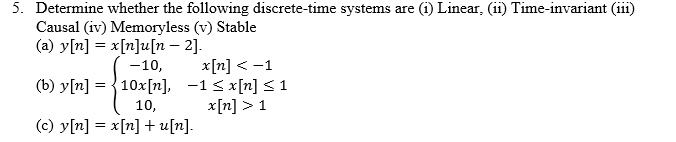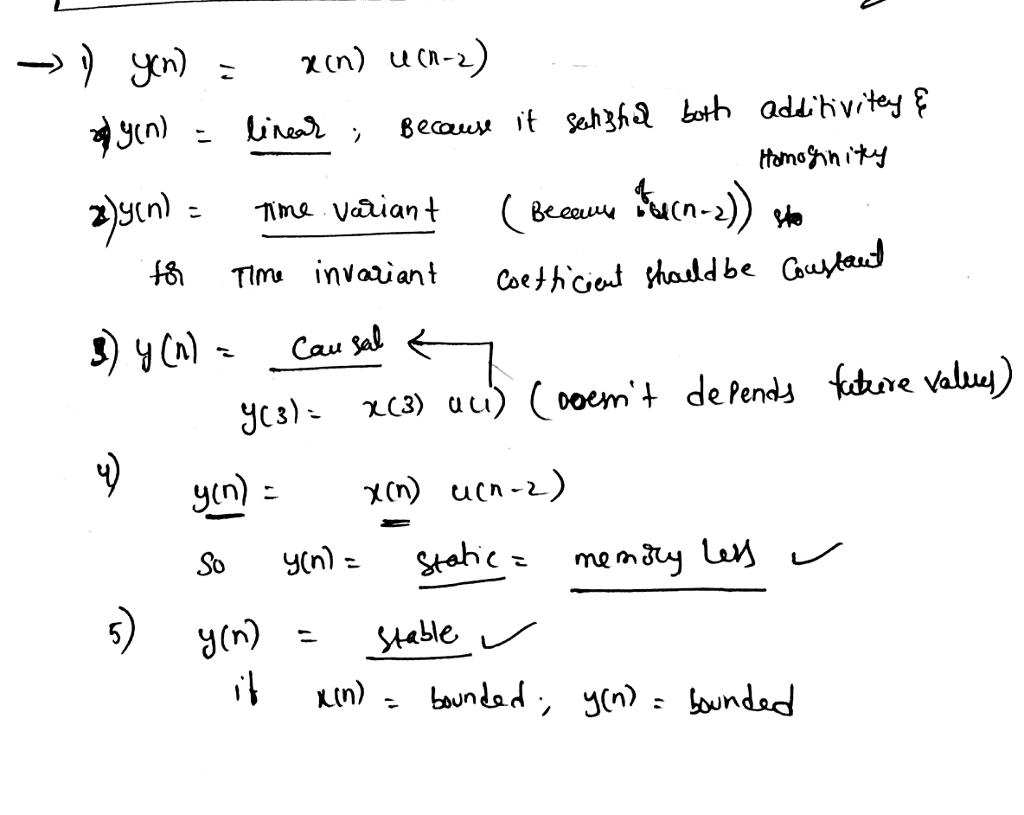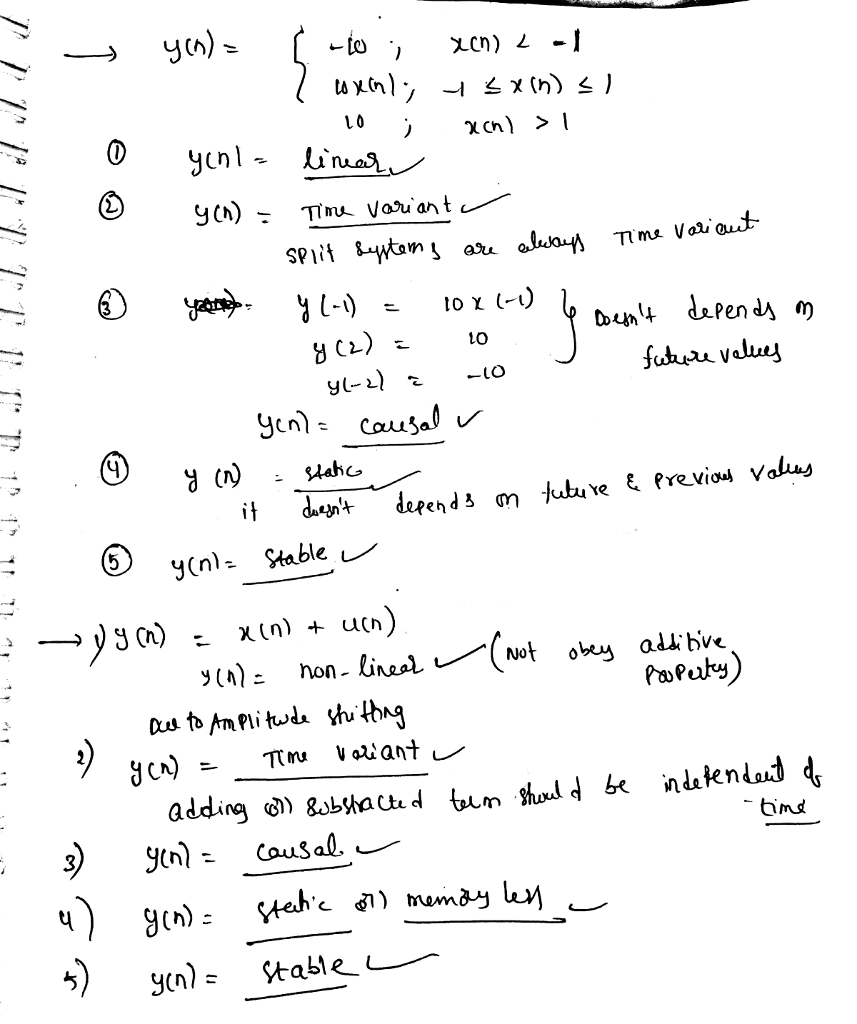# Homework Solution: Determine whether the following discrete-time systems are (i) Linear, (ii) Time-invariant (iii) Ca…Determine whether the following discrete-time systems are (i) Linear, (ii) Time-invariant (iii) Causal (iv) Memoryless (v) Stable (a) y[n] = x[n] u [n - 2]. (b) y[n] = {-10, x[n] 1 (c) y[n] = x[n] + u[n].